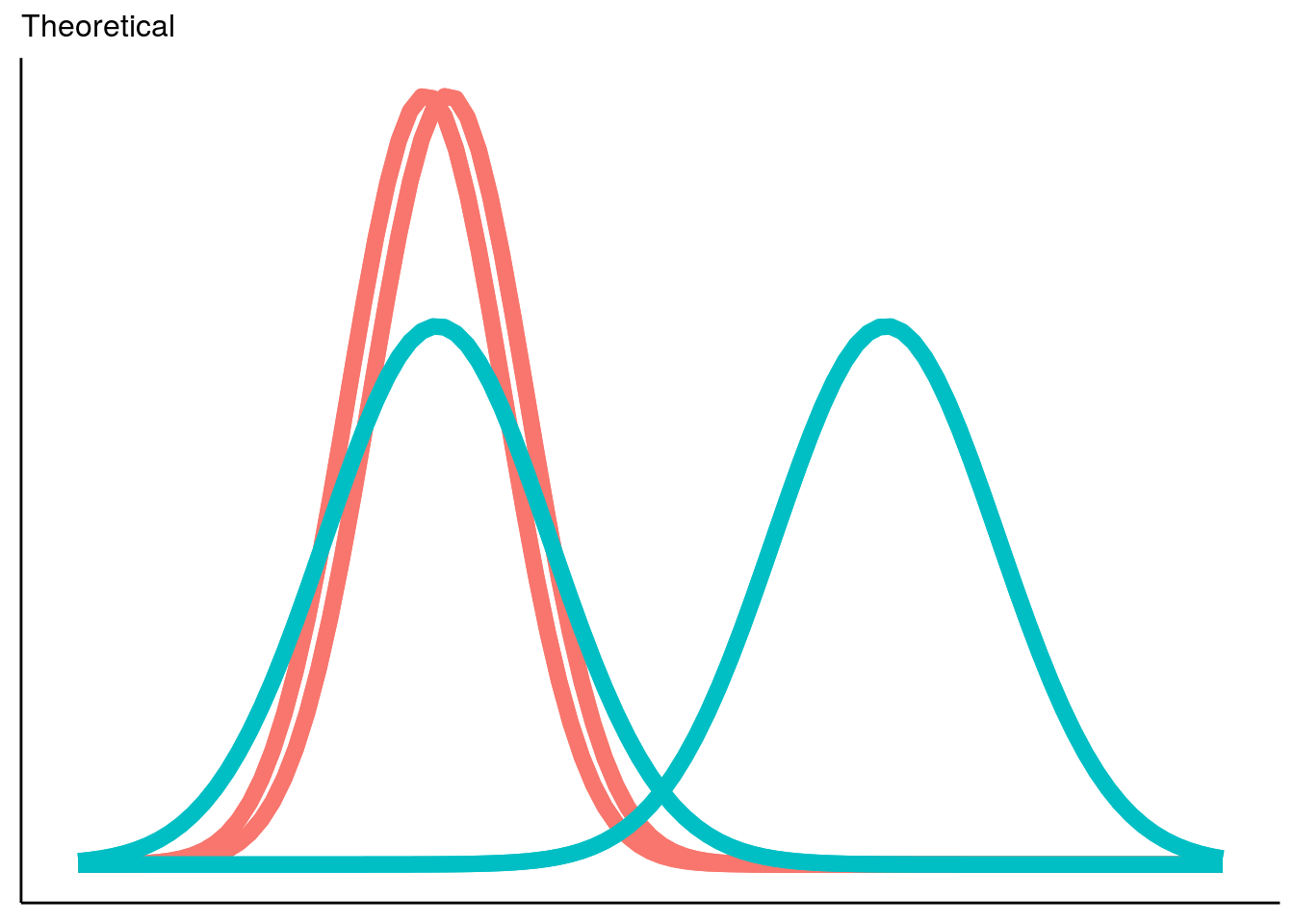## 1. Introduction

### 1.1 Motivation for Developing Oncomix

The advent of large, well-curated databases, such as the genomic data commons, that contain RNA sequencing data from hundreds of patient tumors has made it possible to identify oncogene candidates solely based off of patterns present in mRNA expression data. Oncomix is the first method developed to identify oncogenes in a visually-interpretable manner from RNA-sequencing data in large cohorts of patients.

Oncomix is an R package for identifying oncogene candidates based off of 2-component Gaussian mixture models. It estimates parameters using the expectation maximization procedure as implemented in the R package mclust. This tutorial will demonstrate how to identify oncogene candidates from a set of mRNA sequencing data. We start by loading the package:

``````#devtools::install_github("dpique/oncomix", build_vignettes=T)
library(oncomix)``````

### 1.2 Distribution of Oncogene mRNA Expression

We first explore the idea of what the distribution of gene expression values for a oncogene should look like. It is known that oncogenes such as ERBB2 are overexpressed in 15-20% of all breast cancer patients. In addition, oncogenes should not be expressed in normal tissue. Based on this line of reasoning, we formulate a model for the distribution of oncogene mRNA expression values in a population of both tumor (teal curves) and normal (red-orange curves) tissue:

``````library(ggplot2)
oncoMixIdeal()``````The x-axis represents mRNA expression values, with lower values toward the left and larger values (i.e. higher expression) toward the right. The y axis represents density. The teal curves represent the best-fitting Gaussian probability distribution (PD) over expression values from a single gene obtained from multiple tumor samples. The red-orange curves represent the PD over expression values from the same gene obtained from multiple adjacent normal tissue samples. This mixture model is applied once to the tumor data and again (separately) to the adjacent normal data, hence the 4 curves.

The advantage of applying a 2-component mixture model is that we are able to capture biologically-relevant clusters of gene expression that may naturally exist in the data. Otherwise, we might represent our data with just a single curve sitting in the middle of what really are 2 distinct clusters. Visually, we see that for a theoretical oncogene, there is a subgroup of tumors that overexpresses this gene relative to normal tissue.

### 1.3 Comparison of Oncomix to Existing Differential Expression Methods

We now conceptually compare oncomix to the techniques employed by traditional differential expression analysis (e.g. Student’s t-test, as employed by limma, or DESeq2). These existing approaches make strong assumptions – namely, that the data from a particular group are well-described by distributions with mass concentrated around a central value (such as a ‘mean’). If we were to use one of these approaches on a large dataset, our assumption would be that oncogenes are overexpressed in every tumor sample compared to normal tissue. This assumption can be visualized below:

``oncoMixTraditionalDE()``